Search IntMath
Close

450+ Math Lessons written by Math Professors and Teachers

5 Million+ Students Helped Each Year

1200+ Articles Written by Math Educators and Enthusiasts

Simplifying and Teaching Math for Over 23 Years

# Range hood curved sides problem

By Murray Bourne, 09 Apr 2014

I am a consultant to cabinet manufacturers. I program their cabinet software to calculate and cutout their cabinet parts on CNC (computer numerical control) machines. A lot of cabinet companies create hoods that go over stoves that are curved on both sides and front. I need to calculate the joint of that curve as if it is laying flat. How do you calculate this?

John provided a picture of one of the typical hoods that he is talking about.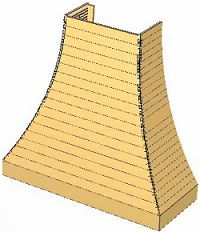What he needs is a formula to plot the Y coordinate of the curve given the X or vice-versa.

This was an interesting problem that involved some actual "real world" applications of math.

The requirement was for a "curved side" with no other specifications, so I proceeded as follows, based on the picture he supplied.

## Solution (1)

I happen to have a Chinese lamp shade which also has curved sides and it's very similar to the image he sent, so I used that as a model to make sure what I had was OK. I like to work with concrete materials first to give me a better feel for what is going on.

I used a piece of paper to outline one side of the shade, then measured the side of the paper (laid flat) in 5 locations and using Cartesian coordinates, it looked like this: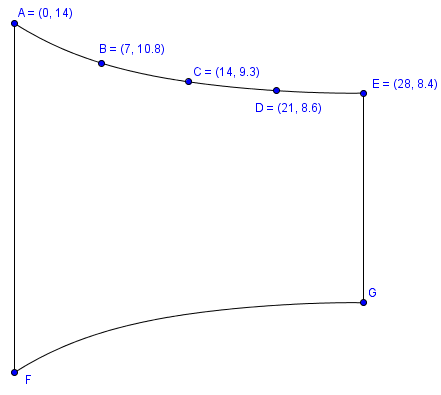For the diagram above, I used my favorite tool for this kind of job, GeoGebra (a free open source tool.)

In Geogebra, it's simple to ask for a polynomial passing through the 5 points, using the following syntax:

Polynomial[A,B,C,D,E]

While it was potentially a quartic (because it passes through 5 points), Geogebra decided a parabola would do the job:

f(x) = 0.03x^2 - 0.64x + 14

NOTE 1: I moved the curve on its side because points AB are almost vertical otherwise, and that gives messy polynomials. It's much smoother if the curve is almost horizontal.

If we had proceeded with the curve in upright mode, the points would have looked like this: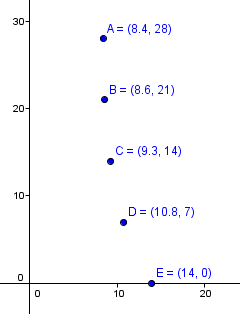With the points almost vertical, the resulting polynomial is a quartic, and certainly not useful to us. (I have changed the vertical scale for this next graph).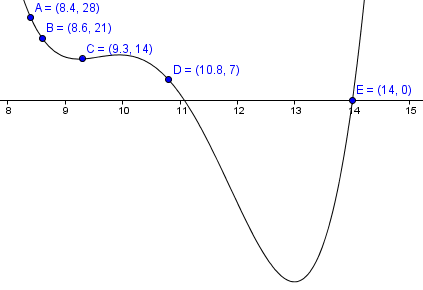We don't get a smooth curve joining the 5 points.

NOTE 2: You can also use Microsoft Excel to find a polynomial for you as well. (See the bottom of the DJIA Graph page for the procedure).

## Back to the problem.

So I printed out my curves on paper, and cut them out. The 4 shapes were like this: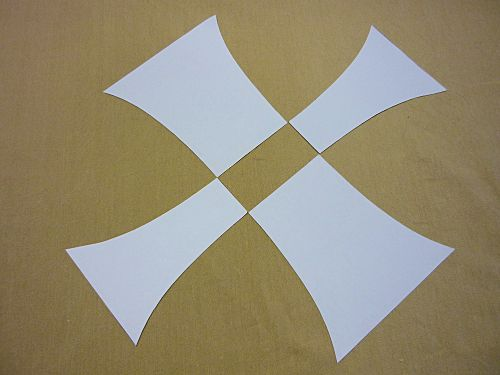And the final mockup (using paper):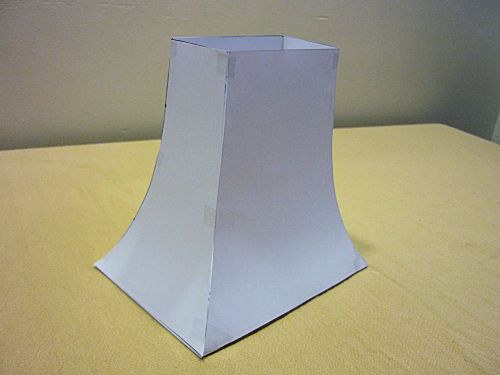Yes, it's rough, but the joins appear to be all good (within the mm or so tolerance of my cutting and sticking...)

So one generic solution for the problem is a parabola:

f(x) = 0.03x^2 - 0.64x + 14

As a side note, this problem reminded me of the shapes of Singapore's iconic Marina Bay Sands Hotel. (I live in Singapore).[Image source]

## Refined problem

John replied to my first solution giving more detailed specifications, as follows:

1. The curves should actually be arcs of a circle (which was not apparent in the first picture John sent)
2. The curved faces will be tangent to the top face of the range hood (that is, it will be vertical at the top of the curve)
3. The curved face is not horizontal at the bottom. It stops before the arc becomes horizontal.

So we now have to figure out the equation of the joins for circular sides, when laid flat.

John also supplied some dimensions for a typical case. It will be 12 units high, and 9.37 units from the top join to the bottom join, in the horizontal direction, as follows: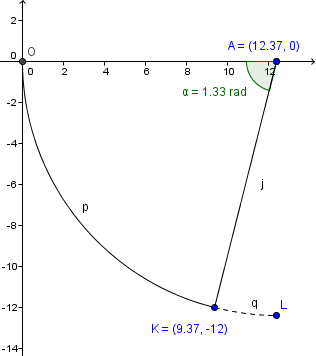The curve at O is vertical, and the arc is 12 units in the vertical direction from O. When we calculate the arc length p, we find it is: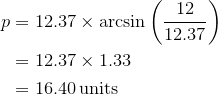This leads us to consider the following possible solutions which represent the curved side laid flat (and once again rotated by 90° for the reasons given above).

Of course, there are infinitely many possible curves passing through (0,0) and (16.4,9.37), but the curve needs to be horizontal at (0,0), so that when we turn it 90°, it will be vertical.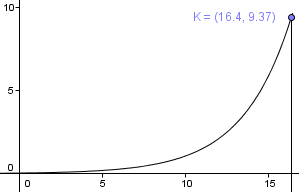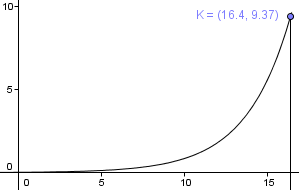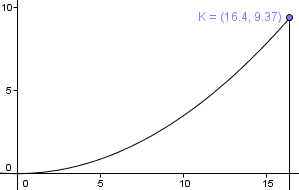Which curve could it be? And, what is the equation of that curve? Remember, it has to fit exactly with the other curves that are at right angles to it, and give us a circular arc when joined.

## Solution (2)

After several failed experiments involving complicated attempts at projecting the curved surface onto a flat plane, I stepped back and observed the 3rd curve looks a lot like a parabola, and the other 2 looked like they weren't going to give us a circular curved surface. The useful paper, Unwrapping Curves from Cylinders and Cones, was the deciding factor.

Assuming now it will be parabolic, and given that it needs to pass through (16.4, 9.37), we can simply use the general form of a parabola: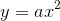Then solve for a by substituting :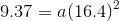This gives us: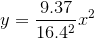I've left it in fraction form so we can more easily see where the general formula comes from later.

I then graphed this from x = 0 to x = 16.4 (the arc length).

Based on the simple parabola, I cut out shapes as in the following.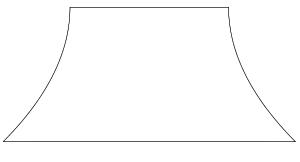Here is the resulting range hood model made from paper.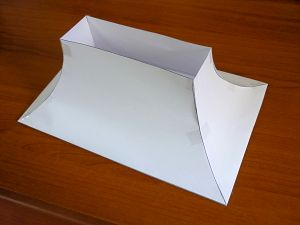The height of the curved part is now 12 units (as required) and the distance from the bottom left point in the picture the the top of the curved join was just over 9 units, as required. (Of course, some tolerance is applied since we are working with paper.)

You can see when we view the model from the side that the curved edges are indeed circular arcs, and it's vertical at the top of the arc.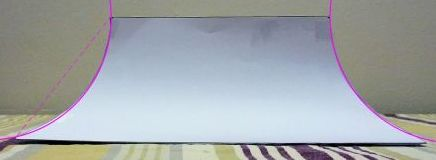## General solution

John wanted a general solution for the curve.

If the radius of the circular arc is given by r, and point K is (m,n) then the arc length will be: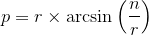The required curve will be:I'll leave it as an exercise for the reader to prove we actually will get sides which are arcs of a circle when using a parabola. ^_^)

## Conclusions

(1) Often the simplest answer is the best.

(2) The quadratic curve is very handy in math!

### 13 Comments on “Range hood curved sides problem”

1. Alan Cooper says:

If it was the profile curves that were circular (which would give the sheets a more easily manufactured constant curvature) then x would just be the arc length and the formula would be y=r(1-cos(x/r)) whose first non-zero Maclaurin term matches your answer. But then the corner curves would be ellipses. To make the corner curves circular we need the cross section curves to be elliptical and with x being the arc length on an ellipse seems to bring in elliptic functions. Does it really work out to be exactly quadratic in that case?

2. Murray says:

@Alan: Good to hear from you. Do you mean the corners as viewed from (say) 45° to the curved edge (as opposed to viewing them along the curved edge as I did in the final photo)?

I love 3-D geometry - it nicely pushes higher-order thinking!

3. Thomas Buckley says:

The resultant shape is part of a Sine wave, so the solution should be a function of a Sine, (or Cosine).
One form is,

y = r[1 + Sin(4.7124 + x/r)] . . Eq1 . . General Solution

Using your values, which are correct, for r = 12.3691,
and a range of x from 0 to arc length p = 16.401.
Note: The Sine of Radians not Degrees is calculated.

Except for the two end points,
your Parabola coordinates are all less than the correct
Sine calculated values and for more than half the 16.401
range the error is greater than 10%! The edges of your
pieces will join well, but will only be near arcs of circles. Practically it is easier to roll metal arcs.

For ANY Stove Hood with curved Height H, and curved Depth D;

Cylinder Radius r = (H^2 + D^2)/2D . . Eq2

And the required Length of arc p = 2rArcTan(D/H) . . Eq3

Make sketches to assist visualization of the following.
Eq1 developed from,
y = hSinθ Where h is the +/- Max or peak value of sinewave.
y = rSinθ . . For a 45 degree cut Sine peaks, h = r
= rSin X/r . . Relative to x-axis. X/r is a Radian value.
. . . . . here X is the arc length from 0
= r + rSin X/r . . Relative to negative peak of sine wave.
. .Observe that a 45 degree miter joint of two cylinders at
. . . . right angles, the required sine curve STARTS . . . . at the MINIMUM ie 270 degrees or at arc 3(PI)r/2
. . . (Calculating from this Sine point is the key to the
. . understanding and development of the following equation)
. . . and continues to 3(PI)r/2 + 16.401
y = r + rSin[{3(PI)r/2 + x)}/r}] . x now extra to 3(PI)r/2
y = r + rSin{3(PI)/2 + x/r} . . 3(PI)/2 = 4.7124

y = r[1 + Sin(4.7124 + x/r)] . Eq1 the GENERAL solution.

Eq2 determines r, and
Eq3 determines p the range for x, 0<=x<=p

Application of the three equations allows any similar hood to be correctly designed.

What's the 4.7124 about? It's the Radian value of 270 deg, the point of minimum value of a Sine wave, our starting point for calculating the required curve.

Thank you for the puzzles, and good luck.

4. R. Grothmann says:

I like this kind of problems and your handling. I think I solved this in my blog. The solutions is not a formula to be liked.

http://observations.rene-grothmann.de/a-construction/

Yours

5. R. Grothmann says:

I see, that I did not solve your problem. I just solved the problem to draw this in 3D.

6. Murray says:

@R. Grothman: No matter - your solution adds to the body of knowledge on this! Thanks for sharing.

7. John says:

Hello All,
I want to thank everyone for all the help on this one. I have worked on this problem for 5 years trying to find a mathematical solution for laying these out in the software we cabinet makers use. I couldn't find anyone to help until I contacted Murray. A couple of things I need to ask.
1) How would the equation change if the angle isn't 45 degrees?
Sometimes the distance from the side is greater than the distance from the front. For instance we have a hood with overall size of 48in wide x 42in tall x 24in deep. The top section of the hood needs to be 24in wide x 10in deep. These sizes are dictated by the customer. So this would make the distance for face as 7in horizontally from the top to the bottom (9.37 above) and the ends as 12in horizontally from the top to the bottom, with the same height. Simple trig tells us that the angle would be ATAN(7/12) or 30.2564 degrees for the one and 59.7435 degrees for the other piece.

I am thinking that the 1 in the equations above would change to the radian value of the corresponding angle? Again I would like to thank all that are helping with this. I finally see the light at the end of a VERY long tunnel.

John

8. Maggie says:

Wow, this post really makes you think deeply about the shape of the range hood. Thank you for giving such a detailed explanation.

9. AARON F ANGELL says:

I too have been on the same quest for solutions to a semi parametric solutions to the pipe size (top variables) and the hood inserts (bottom variables) and the ceiling height variables.

I hate to sound ignorant, but anyway you can interpret this for me?

10. Murray says:

@Aaron: Which part are you not sure about?

11. AARON F ANGELL says:

Sorry, I just reread my comment and I wasn't sure what I meant. lol

so we have standard sizes of these range hoods and through trial and error nailed down the math for the geometry to cut on our cnc machine

so here is my question. is there a way that I could set up some variables in order to derive the math for custom sizes?

I would know the following in almost all cases of the original drawing

height of hood

the bottom width of the hood

width at top of the hood

bottom depth of hood from wall

top depth of hood from wall

I theorized that my arc length at the hip would have to be the same a

every roofing subject on the matter avoids the mathematical solution and advises to lay one or the other out and try to scribe them together.

12. Murray says:

@Aaron: I believe Thomas' approach (Comment #3) is the most likely to produce the kind of calculations you are looking for.

Perhaps if you supply the dimensions for one of the hoods you are talking about, hopefully he will have some time to ponder it. I'm afraid right now I can't promise to do so.

13. Ganga Singh says:

I have been using Thomas's approach for 2 years now and it works efficiently. But we are facing the issue of the bell curve. We are still using the old frame based approach for them. Is there any way to create bell curve in the similar way as a part of a parabola or a sine wave.

### Comment Preview

HTML: You can use simple tags like <b>, <a href="...">, etc.

To enter math, you can can either:

1. Use simple calculator-like input in the following format (surround your math in backticks, or qq on tablet or phone):
a^2 = sqrt(b^2 + c^2)
(See more on ASCIIMath syntax); or
2. Use simple LaTeX in the following format. Surround your math with $$ and $$.
$$\int g dx = \sqrt{\frac{a}{b}}$$
(This is standard simple LaTeX.)

NOTE: You can mix both types of math entry in your comment.